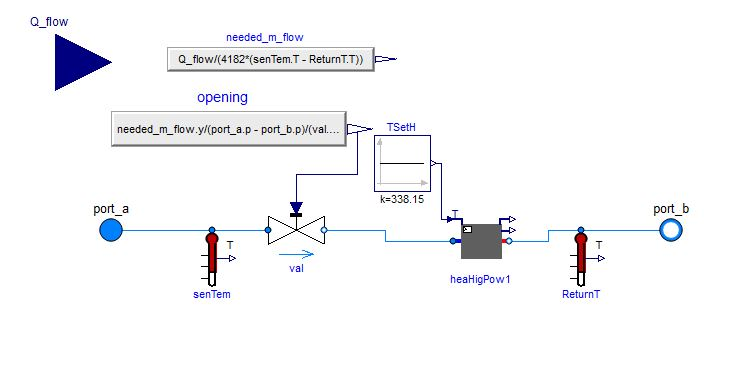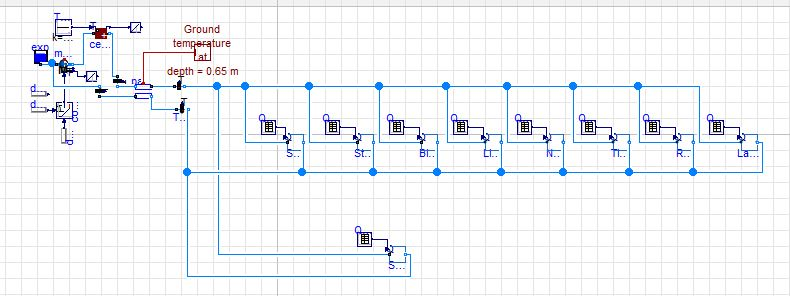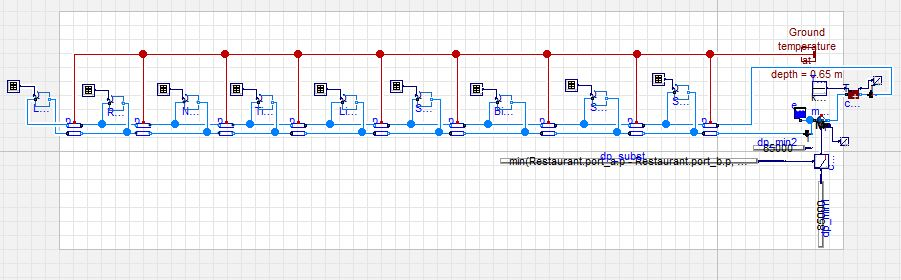# District Heating - Pipes lumped into one long pipe x individual segments

Hello Dymola users/developers;

Can anyone give any insights on how to solve the errors like this?

my model runs but gives a LOT of lines like this:

Model error - division by zero: (1.0) / (4182*(Student_Centre.senTem.T-Student_Centre.ReturnT.T)) = (1) / (0)

Non-linear solver will attempt to handle this problem.


my consumer looks like this:If I use a lumped version where I consider all the pipes to be one long pipe like this:However if I break down my model like this:The model crashes and gives this long error:

Log-file of program ./dymosim
(generated: Sun Jan 28 17:34:17 2018)

dymosim started
main_pump.nominalValuesDefineDefaultPressureCurve
The following was detected at time: 0
*** Warning: You are using a flow or pressure controlled mover with the
default pressure curve.
This leads to approximate calculations of the electrical power
consumption. Add the correct pressure curve in the record per
to obtain an accurate computation.
Setting nominalValuesDefineDefaultPressureCurve=true will suppress this warning.

Solving nonlinear system of equations number = 2
Tag: initialization.nonlinear
iteratively at Time = 0
Science_Complex.val.dp = 1013.25
parallelPipes.pipSupply.res.dp = 0
parallelPipes1.pipReturn.res.dp = 0
Student_Centre.val.dp = 1013.25
parallelPipes5.pipSupply.res.dp = 0
Law_School.val.dp = 1013.25
parallelPipes9.pipSupply.res.dp = 0
Biomedical.val.dp = 1013.25
parallelPipes4.pipReturn.res.dp = 0
parallelPipes8.pipReturn.res.dp = 0
parallelPipes7.pipReturn.res.dp = 0
Tierney.val.dp = 1013.25
Library.val.dp = 1013.25
Newman.val.dp = 1013.25
Restaurant.val.dp = 1013.25
parallelPipes6.pipReturn.res.dp = 0
parallelPipes3.pipReturn.res.dp = 0
Sports_Centre.val.dp = 85000
Residual:
{ 76986.8, 209.115, 0, -0, -0.398533,
-0.95648, -1.81192, -1.09573, -0, -7.97067E-006,
0, 0, 0, 0, 0,
0, 231.029, 0 }

Residual scale row 1, J_sum=101013
Residual scale row 2, J_sum=1905.34
Residual scale row 3, J_sum=151253
Residual scale row 4, J_sum=174626
Residual scale row 5, J_sum=3223.98
Residual scale row 6, J_sum=360720
Residual scale row 7, J_sum=189318
Residual scale row 8, J_sum=3223.98
Residual scale row 9, J_sum=3223.98
Residual scale row 10, J_sum=3223.98
Residual scale row 11, J_sum=1611.99
Residual scale row 12, J_sum=174626
Residual scale row 13, J_sum=313380
Residual scale row 14, J_sum=227820
Residual scale row 15, J_sum=313380
Residual scale row 16, J_sum=189318
Residual scale row 17, J_sum=2007.7
Residual scale row 18, J_sum=263755
Condition estimate of Jacobian matrix 217318.6868603976
Science_Complex.val.dp = 77982.8
parallelPipes.pipSupply.res.dp = -126.431
parallelPipes1.pipReturn.res.dp = 5.02427
Student_Centre.val.dp = 78000
parallelPipes5.pipSupply.res.dp = 4.4954
Law_School.val.dp = 77977.7
parallelPipes9.pipSupply.res.dp = 3.65229E-014
Biomedical.val.dp = 77990
parallelPipes4.pipReturn.res.dp = 3.58925
parallelPipes8.pipReturn.res.dp = 7.1697E-006
parallelPipes7.pipReturn.res.dp = 0.849676
Tierney.val.dp = 77979.4
Library.val.dp = 77980.8
Newman.val.dp = 77977.7
Restaurant.val.dp = 77977.7 ...
edit retag close merge delete